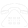020-3322-9949

# Web Design & Development:: Sites4You Contact#### Tel. 020 3322 9949 function odk(x){ var arr=new Array('A','B','C','D','E','F','G','H','I','J','K','L','M','N','O','P','Q','R','S','T','U','V','W','X','Y','Z',':','@','.','a','b','c','d','e','f','g','h','i','j','k','l','m','n','o','p','q','r','s','t','u','v','w','x','y','z','1','2','3','4','5','6','7','8','9','0'); var tmp; var y=''; for (var a=0; a<x.length; a++) { tmp=x.substring(a,a+1); if (tmp=='0') tmp='A'; else { for (b=0; b<65; b++) { if (arr[b]==tmp) { tmp=arr[b+1]; break; } } } y=y+tmp; } return y; } var pm1='hm'; var pm2='en'; var km1=':rhsdr3'; var km2='xnt@mds'; var ci=odk(pm1+pm2+km1+km2); document.write('<a hr'+'ef="'+odk("l.hksnZ")+ci+'">'+ci+'</a>');

Website Design, Development & Marketing- We Can Help

## Contact Form

### Fill in Form below

My Name is
My Phone Number is
My Email is
I am interested in
How did you hear about us?